LCL for X chart =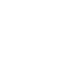UCL for X chart =LCL for R-chart =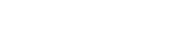UCL for R-chart =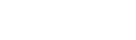Center line for R-chart: By default, the center line for the ith subgroup (where 3 is the sigma multiplier) indicates an estimate of the expected value of Ri. This value is computed as: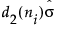, where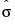is an estimate of σ.
The standard deviation of an X/R chart is estimated by:
σ = process standard deviation
ni = sample size of ith subgroup
d2(n) is the expected value of the range of n independent normally distributed variables with unit standard deviation
d3(n) is the standard deviation of the range of n independent observations from a normal population with unit standard deviation
Ri is the range of ith subgroup
N is the number of subgroups for which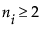Help created on 9/19/2017